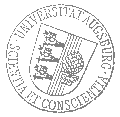# Universität AugsburgInstitut für MathematikOberseminar zur Algebra und Zahlentheorie

Dr. Giordano Cotti
MPIM Bonn

spricht am

Dienstag, 17. April 2018

um

15:45 Uhr

im

Raum 1007 (L1)

über das Thema:

## »Monodromy local moduli of semisimple coalescent Frobenius structures«

 Abstract: Based on a series of joint papers with B. Dubrovin and D. Guzzetti. In occasion of the 1998 ICM in Berlin, B. Dubrovin conjectured an intriguing connection between the enumerative geometry of a Fano manifold X with algebro-geometric properties of exceptional collections in the derived category Db(X). Under the assumption of semisimplicity of the quantum cohomology of X, the conjecture prescribes an explicit form for local invariants of QH∗(X), the so-called monodromy data, in terms of Gram matrices and characteristic classes of objects of exceptional collections. Frobenius manifolds appearing in the study of the conjectural relations mentioned above typically show a coalescence phenomenon at points where the Frobenius algebra is semisimple, but the operator of multiplication by the Euler vector field has not simple spectrum. On the one hand, the definition of monodromy data is based on the analytic theory of isomonodromy deformations, which a priori cannot be applied at coalescence semisimple points of QH∗(X). On the other hand, it turns out that the Frobenius structure may be known only at coalescence points, which are thus the only locus where the monodromy data can actually be computed. This is the case of the small quantum cohomology of complex Grassmannians, for which the occurrence and frequency of the coalescence phenomenon is surprisingly subordinate to the distribution of prime numbers. In this talk I will firstly show how under minimal conditions the classical theory of M. Jimbo, T. Miwa and K. Ueno (1981) can be extended to describe isomonodromy deformations at a coalescing irregular singularity; I will also show how to locally describe the Frobenius structure near coalescing semisimple points. Finally, if time allows, explicit examples will be taken from singularity and quantum cohomology theories.

 Hierzu ergeht herzliche Einladung. Prof. Dr. Maxim Smirnov

Kaffee, Tee und Gebäck eine halbe Stunde vor Vortragsbeginn im Raum 2006 (L1).

[Impressum]      [Datenschutz]      wwwadm@math.uni-augsburg.de,    Di 10-Apr-2018 15:00:14 MESZ